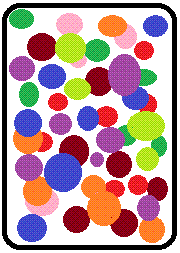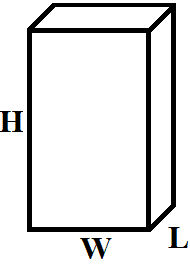If you find any of this useful, please consider donating via PayPal to help keep this site going.# How Much Candy is in the Jar?

2/2/16

We've all played this game, whether in school or at work. You have a jar with candy (or some object; paperclips, bouncy balls, etc.) in it, and the person who comes closest to estimating the correct number of candies in the jar wins a prize.(pretend this is a jar with candy in it!)

Most people, when asked, literally pull a number out of thin air. However, there is a much better way, a way that is logical at least.

First, one assumes the shape of the Jar. From this, one can calculate the volume of the jar, V(J).

For example, if the jar is a "brick" with length L, width W, and height H, then V(J) ~ L*W*HIf the jar is a cylinder with height h and radius r, then V(J) ~ pi*r2*h, and so on, with a different volume equation for different shaped jars.Next, we assume a shape for the Candy.

Calculate the volume of a single candy as V(C). Most candies will probably be approximately spherical. For a sphere with radius s, the volume is V(C) ~ (4/3)pi*s3.

Therefore, the number of candies in the jar ~ V(J)/V(C). For a cylindrical jar and spherical candy, we get number of candies ~ (pi*r2*h) / ((4/3)pi*s3) ~ (3/4)*((r2*h)/s3).

However, this assumes the candies fill the space perfectly, when in reality there is space that is not "filled" by the candies. Consider the discussion at https://en.wikipedia.org/wiki/List_of_shapes_with_known_packing_constant

For candy in the shape of a sphere, the packing constant, K, is K ~ pi/(18.5) ~ 0.7404805.

So a better estimate is, number of candies in the jar ~ .74*(V(J)/V(C)). In the case of a cylindrical jar and spherical candy, this is number of candies ~ .74*(3/4)*((r2*h)/s3).

However, this might not be accurate either. Ask yourself, do the candies look like they are tightly packed, or are they more loosely arranged? See the discussion at https://en.wikipedia.org/wiki/Random_close_pack

Therefore an even better estimate is, number of candies in the jar ~ T*(V(J)/V(C)), where T depends not only on the shape of the candy but also on the tightness of the packing of the candies, and therefore is less than K.

Say the candies are poured in, and we still have a cylindrical jar and spherical candy, then the number of candies in the jar ~ .62*(3/4)*((r2*h)/s3), or .465*((r2*h)/s3).

Another possible estimation method is obtaining the mass of the empty jar Mej, the mass of the full jar Mfj, and the mass of a single candy Mc. Then the number of candies in the jar ~ (Mfj-Mej)/Mc.

To summarize, there are a lot of a factors: the actual shape of the jar, the actual shape of candies (or objects) and how similar all the candies are, and the tightness of the packing of the candies in the jar.

From this way of thinking about the problem, I hope you can see that math, statistics, and logic are more sensible than pure guessing.

If you enjoyed any of my content, please consider supporting it in a variety of ways: